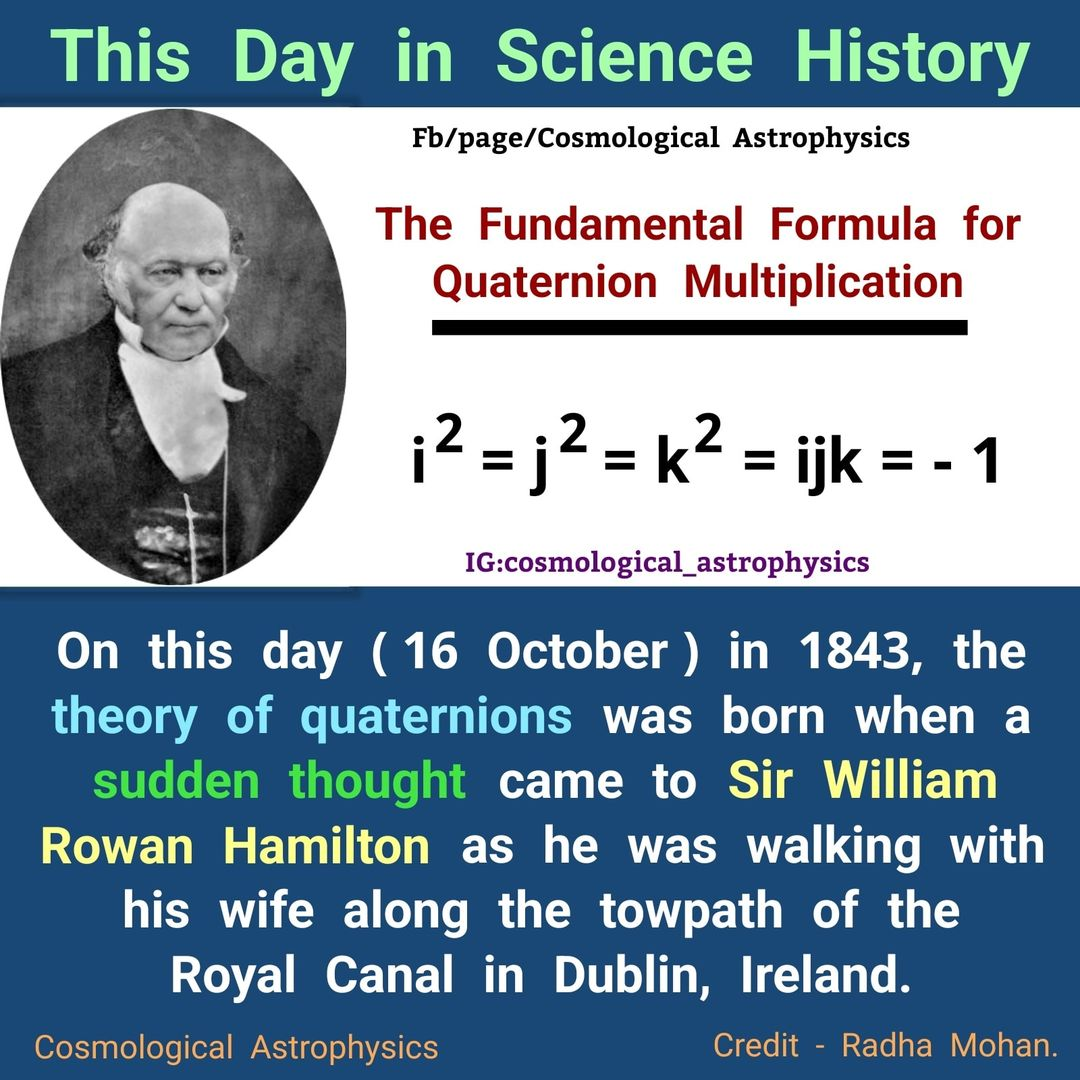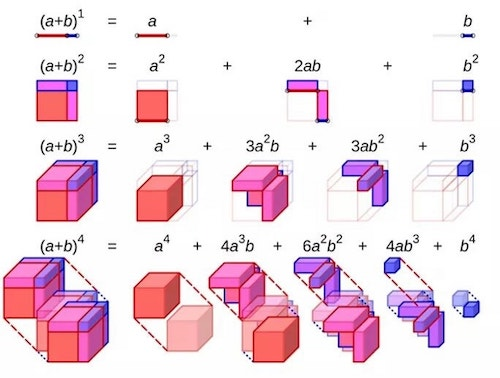# Quaternion

In mathematics, the quaternions are a number system that extends the complex numbers. They were first described by Irish mathematician Sir William Rowan Hamilton in 1843 and applied to mechanics in three-dimensional space. A striking feature of quaternions is that the product of two quaternions is noncommutative, meaning that the product of two quaternions depends on which factor is to the left of the multiplication sign and which factor is to the right.

Hamilton defined a quaternion as the quotient of two directed lines in a three-dimensional space or equivalently as the quotient of two vectors. Quaternions can also be represented as the sum of a scalar and a vector.

Quaternions find uses in both theoretical and applied mathematics, in particular for calculations involving three-dimensional rotations such as in three-dimensional computer graphics and computer vision. They can be used alongside other methods, such as Euler angles and matrices, or as an alternative to them depending on the application.

In modern language, quaternions form a four-dimensional associative normed division algebra over the real numbers, and thus also form a domain. In fact, the quaternions were the first noncommutative division algebra to be discovered. The algebra of quaternions is often denoted by H (for Hamilton), or in blackboard bold by (Unicode U+210D, â„). It can also be given by the Clifford algebra classifications Câ„“0,2(R) = Câ„“03,0(R). The algebra H holds a special place in analysis since, according to the Frobenius theorem, it is one of only two finite-dimensional division rings containing the real numbers as a proper subring, the other being the complex numbers.

The unit quaternions can therefore be thought of as a choice of a group structure on the 3-sphere S3, the group Spin(3), the group SU(2), or the universal cover of SO(3). wikipedia - quaternion

in 1843, the theory of quaternions was born when Sir William Rowan Hamilton was on his way to the Royal Irish Academy where he was going to preside at a council meeting. As he walked along the towpath of the Royal Canal (in Dublin, Ireland) with his wife, the concepts behind quaternions were taking shape in his mind. When the answer dawned on him, Hamilton could not resist the urge to carve the formula for the quaternions into the stone of Brougham Bridge as he paused on it.
He had been working since the late 1830s on the basic principles of algebra, resulting in a theory of conjugate functions (algebraic couples) in which complex numbers are expressed as ordered pairs of real numbers. Until now, he had not been successful in developing a theory of triplets that could be applied to three-dimensional geometric problems. During the walk, he realized that the theory should involve quadruplets, not triplets."Not a single one of the equations universally taught as "Maxwell's equations" ever appeared in any book or paper by James Clerk Maxwell; instead, they are Oliver Heaviside's equations. Maxwell's actual theory was written in quaternions, which is a complete system of mathematics." [Bearden, The Final Secret of Free Energy]Possible Quantum Arithmetic in 3D?

James Clerk Maxwell
"9 coefficients determine the relationship between flux and intensity
6 of these coefficients form 3 pairs of equal quantities
3 pairs of equal coefficients will self-conjugate." [James Clerk Maxwell]

The original quote from "https://svpwiki.com/pdffiles/A_Treatise_on_Electricity_and_Magnetism.pdf - vol. I".

"The case in which the components of the flux are linear functions of those of the force is discussed in the chapter on the Equations of Conduction, Art. 296. There are in general nine coefficients which determine the relation between the force and the flux. In certain cases we have reason to believe that six of these coefficients form three pairs of equal quantities. In such cases the relation between the line of direction of the force and the normal plane of the flux is of the same kind as that between a diameter of an ellipsoid and its conjugate diametral plane. In Quaternion language, the one vector is said to be a linear and vector function of the other, and when there are three pairs of equal coefficients the function is said to be self-conjugate." [James Maxwell, A Treatise on Electricity and Magnetism - vol. I]

Hughes
Among the many subjects which excite interest at the present time is the question whether the doctrine of Evolution is true or false. Milton had evidently some glimpse of its truth, as we see in the following lines:—

"Air and ye elements! the eldest birth
Of Nature's womb, that in quaternion run
Perpetual circle, multiform, and mix
And nourish all things; let your ceaseless change
Vary to our great Maker still new praise!" [Paradise Lost, Book V.] [Harmonies of Tones and Colours, Reflections on the Scheme4, page 46]